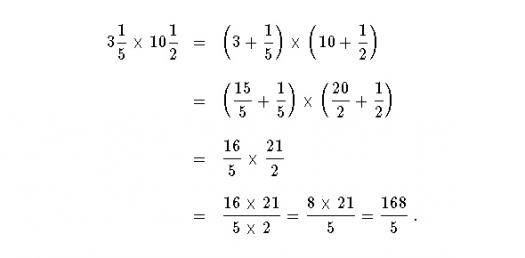# Literal And Compound Fractions Quiz!

Approved & Edited by ProProfs Editorial Team
At ProProfs Quizzes, our dedicated in-house team of experts takes pride in their work. With a sharp eye for detail, they meticulously review each quiz. This ensures that every quiz, taken by over 100 million users, meets our standards of accuracy, clarity, and engagement.
P
Community Contributor
Quizzes Created: 1 | Total Attempts: 687
Questions: 20 | Attempts: 701Settings.

• 1.

### What's the opposite of -2/3 and the reciprocal of 1/5?

• A.

2/3 and 5

• B.

3/2 and -5

• C.

-2 and 5

• D.

-3 and -5

A. 2/3 and 5
Explanation
The opposite of -2/3 is 2/3 because the opposite of a fraction is found by changing the sign of the numerator. The reciprocal of 1/5 is 5 because the reciprocal of a fraction is found by flipping the fraction upside down. Therefore, the opposite of -2/3 is 2/3 and the reciprocal of 1/5 is 5.

Rate this question:

• 2.

• A.

• B.

• C.

• D.

C.
• 3.

• A.

• B.

• C.

• D.

​​​​​​​

A.
• 4.

### Evaluate the numerical value of  where a=4 and b=8

• A.
• B.
• C.
• D.
D.
Explanation
The expression can be evaluated by substituting the given values of a and b into the expression. So, substituting a=4 and b=8, we get 4^3 - 2(8) = 64 - 16 = 48.

Rate this question:

• 5.

• A.

1

• B.

2

• C.

• D.

A. 1
• 6.

### Evaluate the numerical value of  where c=12 and d=-1

• A.
• B.
• C.
• D.
A.
Explanation
The given expression is 12^(-1). When a number is raised to the power of -1, it means taking the reciprocal of that number. Therefore, 12^(-1) is equal to 1/12.

Rate this question:

• 7.

### Evaluate the numerical value of  where e=5 and f=2

• A.

• B.

• C.

• D.

​​​​​​​

C.
Explanation
The numerical value of e^f can be evaluated by raising e to the power of f. In this case, since e=5 and f=2, the calculation would be 5^2, which equals 25.

Rate this question:

• 8.

• A.

3

• B.

• C.

• D.

D.
• 9.

• A.
• B.
• C.
• D.
A.
• 10.

• A.

​​​​​​​

• B.

• C.

• D.

​​​​​​​

C.
• 11.

• A.

• B.

• C.

• D.

D.
• 12.

• A.

• B.

• C.

• D.

A.
• 13.

• A.

• B.

• C.

• D.

B.
• 14.

• A.

• B.
• C.

• D.
C.
• 15.

• A.

• B.

• C.

• D.

D.
• 16.

• A.

• B.

• C.

• D.

C.
• 17.

### Simplify

• A.

• B.

• C.

• D.

4

D. 4
Explanation
The given question is asking to simplify the expression "4". Since "4" is already a simplified form and there are no further operations or simplifications required, the answer remains as "4".

Rate this question:

• 18.

• A.

• B.

• C.

• D.

A.
• 19.

• A.

• B.

• C.

• D.

C.
• 20.

### Simplify

• A.

1

• B.

• C.

2

• D.Back to top